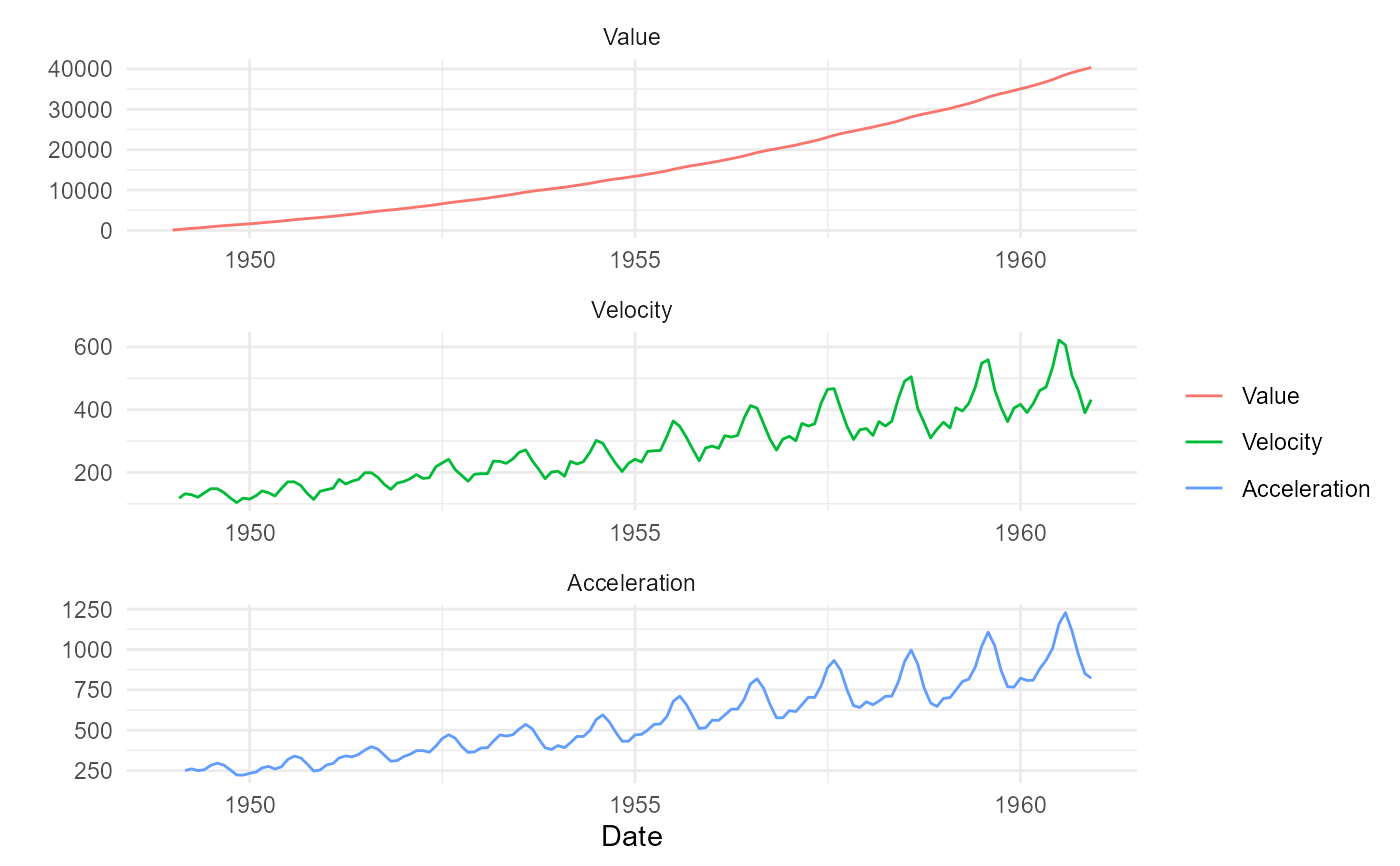This function will produce three plots faceted on a single graph. The three graphs are the following:

• Value Plot (Actual values)

• Value Velocity Plot

• Value Acceleration Plot

## Usage

ts_vva_plot(.data, .date_col, .value_col)

## Arguments

.data

The data you want to visualize. This should be pre-processed and the aggregation should match the .frequency argument.

.date_col

The data column from the .data argument.

.value_col

The value column from the .data argument

## Value

The original time series augmented with the differenced data, a static plot and a plotly plot of the ggplot object. The output is a list that gets returned invisibly.

## Details

This function expects to take in a data.frame/tibble. It will return a list object that contains the augmented data along with a static plot and an interactive plotly plot. It is important that the data be prepared and have at minimum a date column and the value column as they need to be supplied to the function. If your data is a ts, xts, zoo or mts then use ts_to_tbl() to convert it to a tibble.

## Author

Steven P. Sanderson II, MPH

## Examples

suppressPackageStartupMessages(library(dplyr))

data_tbl <- ts_to_tbl(AirPassengers) %>%
select(-index)

ts_vva_plot(data_tbl, date_col, value)$plots$static_plot
#> Warning: Removed 3 rows containing missing values (geom_line()).